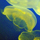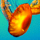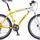## General Question# How do I calculate demand?

Asked by robo (5) February 3rd, 2007
Suppose utility is given by U = ln(x1)+ 2ln(x2), and prices are given by p1 and p2, and income is given by m. a. Solve for the demand for x1 and x2 as a function of price and income. b. What is the price elasticity of demand for x1? What is the income elasticity for x1? c. Use the demand curve to find the utility maximizing values of x1 and x2 separately for each income level m=10, m=20, and m=30 when p1=2 and p2=4. Use these values to help you draw the income offer curve and Engel curves for x1 and x2.
Observing members: 0Composing members: 0I think demand is something like X1(P1, P2, m) and X2(P1, P2, m) and yeah...
calligal000 (16)“Great Answer” (1) Flag as…taxlover (21)“Great Answer” (1) Flag as…1. U'x/U'y=Px/Py --> (1/X1)/(2/X2)=Px/Py --> X2=2Px*X1/Py
taxlover (21)“Great Answer” (0) Flag as…2. m=Px*X+Py*Y
taxlover (21)“Great Answer” (0) Flag as…1,2--> 3. m=Px*X+2Px*X*Py/Py=3Px*X
taxlover (21)“Great Answer” (0) Flag as…X=m/3Px
taxlover (21)“Great Answer” (0) Flag as…Y=2Px*(m/3Px)/py --> Y=2m/3Py
taxlover (21)“Great Answer” (0) Flag as…Y=2Px*(m/3Px)/py --> Y=2m/3Py
taxlover (21)“Great Answer” (0) Flag as…Now - I am a bit rusty, so I may have made a mistake. I must say it seems quite weird that the demands do not depend on the price of the other product. Also, please subsitute X2 for Y.
taxlover (21)“Great Answer” (0) Flag as…If you want me to continue, I will do so - it's getting late on this side of the globe.
taxlover (21)“Great Answer” (0) Flag as…or